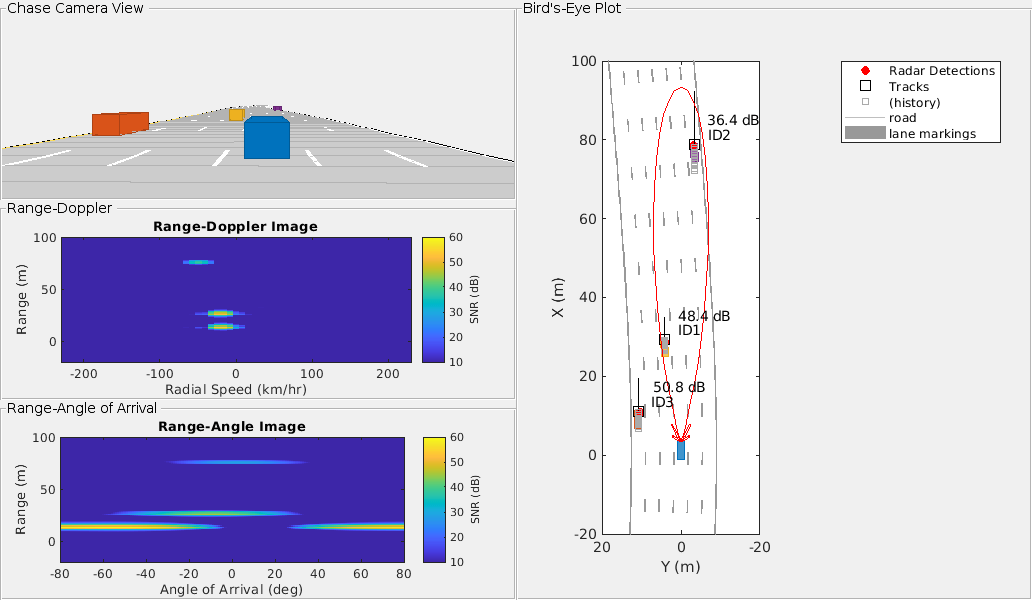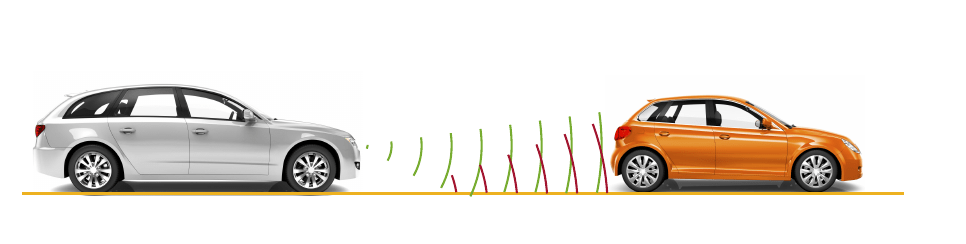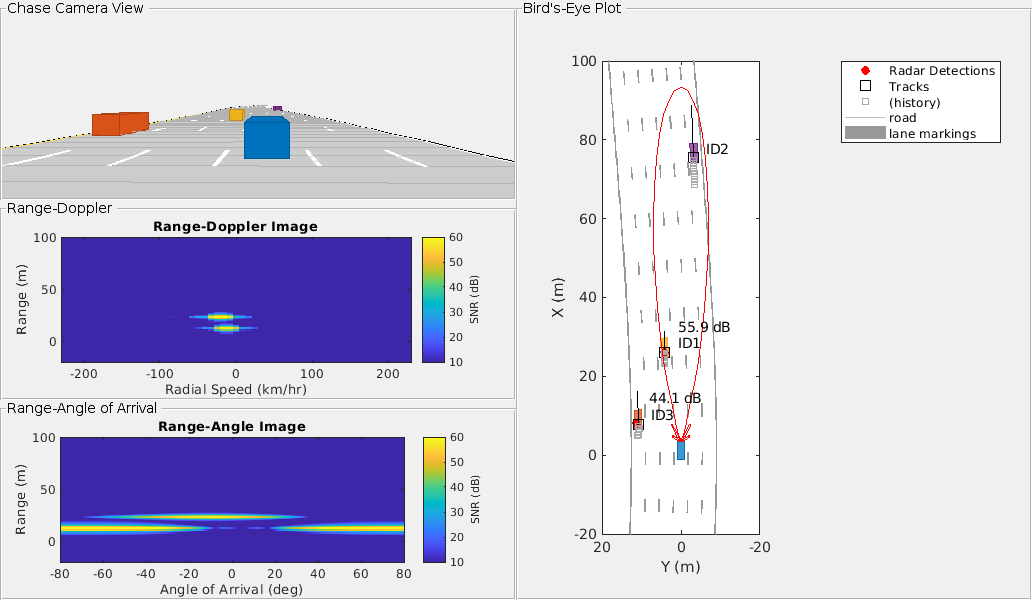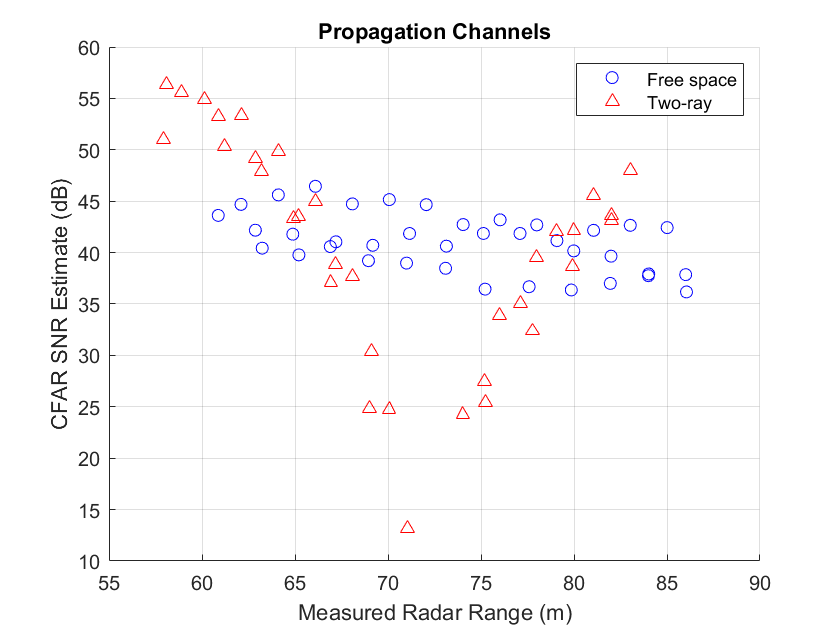# Radar Signal Simulation and Processing for Automated Driving

This example shows how to model a radar's hardware, signal processing, and propagation environment for a driving scenario. First you model a highway scenario using Automated Driving Toolbox™. Then, you develop a model of the radar transmit and receive hardware, signal processing and tracker using Radar Toolbox™. Finally, you simulate multipath propagation effects on the radar model.

### Introduction

You can model vehicle motion by using the `drivingScenario` object from Automated Driving Toolbox. The vehicle ground truth can then be used as an input to the radar model to generate synthetic sensor detections. For an example of this workflow, see Simulate Radar Ghosts due to Multipath Return. The automotive radar used in this example uses a statistical model that is parameterized according to high-level radar specifications. The generic radar architecture modeled in this example does not include specific antenna configurations, waveforms, or unique channel propagation characteristics. When designing an automotive radar, or when a radar's specific architecture is known, using a radar model that includes this additional information is recommended.

Radar Toolbox enables you to evaluate different radar architectures. You can explore different transmit and receive array configurations, waveforms, and signal processing chains. You can also evaluate your designs against different channel models to assess their robustness to different environmental conditions. This modeling helps you to identify the specific design that best fits your application requirements.

In this example, you learn how to define a radar model from a set of system requirements for a long-range radar. You then simulate a driving scenario to generate detections from your radar model. A tracker is used to process these detections to generate precise estimates of the position and velocity of the vehicles detected by your automotive radar.

The radar parameters are defined for the frequency-modulated continuous wave (FMCW) waveform, as described in the example Automotive Adaptive Cruise Control Using FMCW Technology. The radar operates at a center frequency of 77 GHz. This frequency is commonly used by automotive radars. For long-range operation, the radar must detect vehicles at a maximum range of 250-300 meters in front of the ego vehicle. The radar is required to resolve objects in range that are at least 1 meter apart. Because this is a forward-facing radar application, the radar also needs to handle targets with large closing speeds, as high as 230 km/hr.

The radar is designed to use an FMCW waveform. These waveforms are common in automotive applications because they enable range and Doppler estimation through computationally efficient FFT operations. For illustration purpose, in this example, the radar is configured to a maximum range of 100 meters.

```% Set random number generator for repeatable results rng(2017); % Compute hardware parameters from specified long-range requirements fc = 77e9; % Center frequency (Hz) c = physconst('LightSpeed'); % Speed of light in air (m/s) lambda = freq2wavelen(fc,c); % Wavelength (m) % Set the chirp duration to be 5 times the max range requirement rangeMax = 100; % Maximum range (m) tm = 5*range2time(rangeMax,c); % Chirp duration (s) % Determine the waveform bandwidth from the required range resolution rangeRes = 1; % Desired range resolution (m) bw = range2bw(rangeRes,c); % Corresponding bandwidth (Hz) % Set the sampling rate to satisfy both the range and velocity requirements % for the radar sweepSlope = bw/tm; % FMCW sweep slope (Hz/s) fbeatMax = range2beat(rangeMax,sweepSlope,c); % Maximum beat frequency (Hz) vMax = 230*1000/3600; % Maximum Velocity of cars (m/s) fdopMax = speed2dop(2*vMax,lambda); % Maximum Doppler shift (Hz) fifMax = fbeatMax+fdopMax; % Maximum received IF (Hz) fs = max(2*fifMax,bw); % Sampling rate (Hz) % Configure the FMCW waveform using the waveform parameters derived from % the long-range requirements waveform = phased.FMCWWaveform('SweepTime',tm,'SweepBandwidth',bw,... 'SampleRate',fs,'SweepDirection','Up'); if strcmp(waveform.SweepDirection,'Down') sweepSlope = -sweepSlope; end Nsweep = 192; sig = waveform();```

The radar uses an isotropic element to transmit and a uniform linear array (ULA) to receive the radar waveforms. Using a linear array enables the radar to estimate the azimuthal direction of the reflected energy received from the target vehicles. The long-range radar needs to detect targets across a coverage area that spans 15 degrees in front of the ego vehicle. A 6-element receive array satisfies this requirement by providing a 17-degree half-power beamwidth. On transmit, the radar uses only a single array element, enabling it to cover a larger area than on receive.

```% Model the antenna element antElmnt = phased.IsotropicAntennaElement('BackBaffled',true); % Construct the receive array Ne = 6; rxArray = phased.ULA('Element',antElmnt,'NumElements',Ne,... 'ElementSpacing',lambda/2); % Half-power beamwidth of the receive array hpbw = beamwidth(rxArray,fc,'PropagationSpeed',c)```
```hpbw = 17.1800 ```

Model the radar transmitter for a single transmit channel, and model a receiver preamplifier for each receive channel, using the parameters defined in the example Automotive Adaptive Cruise Control Using FMCW Technology.

```antAperture = 6.06e-4; % Antenna aperture (m^2) antGain = aperture2gain(antAperture,lambda); % Antenna gain (dB) txPkPower = db2pow(5)*1e-3; % Tx peak power (W) txGain = antGain; % Tx antenna gain (dB) rxGain = antGain; % Rx antenna gain (dB) rxNF = 4.5; % Receiver noise figure (dB) % Waveform transmitter transmitter = phased.Transmitter('PeakPower',txPkPower,'Gain',txGain); % Radiator for single transmit element radiator = phased.Radiator('Sensor',antElmnt,'OperatingFrequency',fc); % Collector for receive array collector = phased.Collector('Sensor',rxArray,'OperatingFrequency',fc); % Receiver preamplifier receiver = phased.ReceiverPreamp('Gain',rxGain,'NoiseFigure',rxNF,... 'SampleRate',fs); % Define radar radar = radarTransceiver('Waveform',waveform,'Transmitter',transmitter,... 'TransmitAntenna',radiator,'ReceiveAntenna',collector,'Receiver',receiver); ```

### Define Radar Signal Processing Chain

The radar collects multiple sweeps of the waveform on each of the linear phased array antenna elements. These collected sweeps form a data cube, which is defined in Radar Data Cube. These sweeps are coherently processed along the fast- and slow-time dimensions of the data cube to estimate the range and Doppler of the vehicles.

Estimate the direction-of-arrival of the received signals using a root MUSIC estimator. A beamscan is also used for illustrative purposes to help visualize the spatial distribution of the received signal energy.

```% Direction-of-arrival estimator for linear phased array signals doaest = phased.RootMUSICEstimator(... 'SensorArray',rxArray,... 'PropagationSpeed',c,'OperatingFrequency',fc,... 'NumSignalsSource','Property','NumSignals',1); % Scan beams in front of ego vehicle for range-angle image display angscan = -80:80; beamscan = phased.PhaseShiftBeamformer('Direction',[angscan;0*angscan],... 'SensorArray',rxArray,'OperatingFrequency',fc); % Form forward-facing beam to detect objects in front of the ego vehicle beamformer = phased.PhaseShiftBeamformer('SensorArray',rxArray,... 'PropagationSpeed',c,'OperatingFrequency',fc,'Direction',[0;0]); ```

Use the `phased.RangeDopplerResponse` object to perform the range and Doppler processing on the radar data cubes. Use a Hanning window to suppress the large sidelobes produced by the vehicles when they are close to the radar.

```Nft = waveform.SweepTime*waveform.SampleRate; % Number of fast-time samples Nst = Nsweep; % Number of slow-time samples Nr = 2^nextpow2(Nft); % Number of range samples Nd = 2^nextpow2(Nst); % Number of Doppler samples rngdopresp = phased.RangeDopplerResponse('RangeMethod','FFT',... 'DopplerOutput','Speed','SweepSlope',sweepSlope,... 'RangeFFTLengthSource','Property','RangeFFTLength',Nr,... 'RangeWindow','Hann',... 'DopplerFFTLengthSource','Property','DopplerFFTLength',Nd,... 'DopplerWindow','Hann',... 'PropagationSpeed',c,'OperatingFrequency',fc,'SampleRate',fs);```

Identify detections in the processed range and Doppler data by using a constant false alarm rate (CFAR) detector. CFAR detectors estimate the background noise level of the received radar data. Detections are found at locations where the signal power exceeds the estimated noise floor by a certain threshold. Low threshold values result in a higher number of reported false detections due to environmental noise. Increasing the threshold produces fewer false detections, but also reduces the probability of detection of an actual target in the scenario. For more information on CFAR detection, see the example .

```% Guard cell and training regions for range dimension nGuardRng = 4; nTrainRng = 4; nCUTRng = 1+nGuardRng+nTrainRng; % Guard cell and training regions for Doppler dimension dopOver = round(Nd/Nsweep); nGuardDop = 4*dopOver; nTrainDop = 4*dopOver; nCUTDop = 1+nGuardDop+nTrainDop; cfar = phased.CFARDetector2D('GuardBandSize',[nGuardRng nGuardDop],... 'TrainingBandSize',[nTrainRng nTrainDop],... 'ThresholdFactor','Custom','CustomThresholdFactor',db2pow(13),... 'NoisePowerOutputPort',true,'OutputFormat','Detection index'); % Perform CFAR processing over all of the range and Doppler cells freqs = ((0:Nr-1)'/Nr-0.5)*fs; rnggrid = beat2range(freqs,sweepSlope); iRngCUT = find(rnggrid>0); iRngCUT = iRngCUT((iRngCUT>=nCUTRng)&(iRngCUT<=Nr-nCUTRng+1)); iDopCUT = nCUTDop:(Nd-nCUTDop+1); [iRng,iDop] = meshgrid(iRngCUT,iDopCUT); idxCFAR = [iRng(:) iDop(:)]'; % Perform clustering algorithm to group detections clusterer = clusterDBSCAN('Epsilon',2);```

The `phased.RangeEstimator` and `phased.DopplerEstimator` objects convert the locations of the detections found in the range-Doppler data into measurements and their corresponding measurement variances. These estimators fit quadratic curves to the range-Doppler data to estimate the peak location of each detection. The resulting measurement resolutions are a fraction of the range and Doppler sampling of the data.

The root-mean-square (RMS) range resolution of the transmitted waveform is needed to compute the variance of the range measurements. The Rayleigh range resolution for the long-range radar was defined previously as 1 meter. The Rayleigh resolution is the minimum separation at which two unique targets can be resolved. This value defines the distance between range resolution cells for the radar. However, the variance of the target within a resolution cell is determined by the waveform's RMS resolution. For an LFM chirp waveform, the relationship between the Rayleigh resolution and the RMS resolution is given by .

`${\sigma }_{RMS}=\sqrt{12}{\Delta }_{Rayleigh}$`

where ${\sigma }_{RMS}$ is the RMS range resolution and ${\Delta }_{Rayleigh}$ is the Rayleigh range resolution.

The variance of the Doppler measurements depends on the number of sweeps processed.

Now, create the range and Doppler estimation objects using the parameters previously defined.

```rmsRng = sqrt(12)*rangeRes; rngestimator = phased.RangeEstimator('ClusterInputPort',true,... 'VarianceOutputPort',true,'NoisePowerSource','Input port',... 'RMSResolution',rmsRng); dopestimator = phased.DopplerEstimator('ClusterInputPort',true,... 'VarianceOutputPort',true,'NoisePowerSource','Input port',... 'NumPulses',Nsweep);```

To further improve the precision of the estimated vehicle locations, pass the radar's detections to a tracker. Configure the tracker to use an extended Kalman filter (EKF), which converts the spherical radar measurements into the ego vehicle's Cartesian coordinate frame. Also configure the tracker to use constant velocity dynamics for the detected vehicles. By comparing vehicle detections over multiple measurement time intervals, the tracker further improves the accuracy of the vehicle positions and provides vehicle velocity estimates.

```tracker = radarTracker('FilterInitializationFcn',@initcvekf,... 'AssignmentThreshold',50);```

### Model Free Space Propagation Channel

Use the free space channel to model the propagation of the transmitted and received radar signals.

In a free space model, the radar energy propagates along a direct line-of-sight between the radar and the target vehicles, as shown in the following illustration.### Simulate the Driving Scenario

Create a highway driving scenario with three vehicles traveling in the vicinity of the ego vehicle. The vehicles are modeled as cuboids, and have different velocities and positions defined in the driving scenario. The ego vehicle is moving with a velocity of 80 km/hr and the other three cars are moving at 110 km/hr, 100 km/hr, and 130 km/hr respectively. For details on modeling a driving scenario see the example . The radar sensor is mounted on the front of the ego vehicle.

To create the driving scenario, use the `helperAutoDrivingRadarSigProc` function. To examine the contents of this function, use the `edit('helperAutoDrivingRadarSigProc')` command.

```% Create driving scenario [scenario,egoCar,radarParams] = ... helperAutoDrivingRadarSigProc('Setup Scenario',c,fc);```

The following loop uses the `drivingScenario` object to advance the vehicles in the scenario. At every simulation time step, a radar data cube is assembled by collecting 192 sweeps of the radar waveform. The assembled data cube is then processed in range and Doppler. The range and Doppler processed data is then beamformed, and CFAR detection is performed on the beamformed data. Range, radial speed, and direction of arrival measurements are estimated for the CFAR detections. These detections are then assembled into `objectDetection` objects, which are then processed by the `radarTracker` object.

```% Initialize display for driving scenario example helperAutoDrivingRadarSigProc('Initialize Display',egoCar,radarParams,... rxArray,fc,vMax,rangeMax); tgtProfiles = actorProfiles(scenario); tgtProfiles = tgtProfiles(2:end); tgtHeight = [tgtProfiles.Height]; % Run the simulation loop sweepTime = waveform.SweepTime; while advance(scenario) % Get the current scenario time time = scenario.SimulationTime; % Get current target poses in ego vehicle's reference frame tgtPoses = targetPoses(egoCar); tgtPos = reshape([tgtPoses.Position],3,[]); % Position point targets at half of each target's height tgtPos(3,:) = tgtPos(3,:)+0.5*tgtHeight; tgtVel = reshape([tgtPoses.Velocity],3,[]); % Assemble data cube at current scenario time Xcube = zeros(Nft,Ne,Nsweep); for m = 1:Nsweep ntgt = size(tgtPos,2); tgtStruct = struct('Position',mat2cell(tgtPos(:).',1,repmat(3,1,ntgt)),... 'Velocity',mat2cell(tgtVel(:).',1,repmat(3,1,ntgt)),... 'Signature',{rcsSignature,rcsSignature,rcsSignature}); rxsig = radar(tgtStruct,time+(m-1)*sweepTime); % Dechirp the received signal rxsig = dechirp(rxsig,sig); % Save sweep to data cube Xcube(:,:,m) = rxsig; % Move targets forward in time for next sweep tgtPos = tgtPos+tgtVel*sweepTime; end % Calculate the range-Doppler response [Xrngdop,rnggrid,dopgrid] = rngdopresp(Xcube); % Beamform received data Xbf = permute(Xrngdop,[1 3 2]); Xbf = reshape(Xbf,Nr*Nd,Ne); Xbf = beamformer(Xbf); Xbf = reshape(Xbf,Nr,Nd); % Detect targets Xpow = abs(Xbf).^2; [detidx,noisepwr] = cfar(Xpow,idxCFAR); % Cluster detections [~,clusterIDs] = clusterer(detidx.'); % Estimate azimuth, range, and radial speed measurements [azest,azvar,snrdB] = ... helperAutoDrivingRadarSigProc('Estimate Angle',doaest,... conj(Xrngdop),Xbf,detidx,noisepwr,clusterIDs); azvar = azvar+radarParams.RMSBias(1)^2; [rngest,rngvar] = rngestimator(Xbf,rnggrid,detidx,noisepwr,clusterIDs); rngvar = rngvar+radarParams.RMSBias(2)^2; [rsest,rsvar] = dopestimator(Xbf,dopgrid,detidx,noisepwr,clusterIDs); % Convert radial speed to range rate for use by the tracker rrest = -rsest; rrvar = rsvar; rrvar = rrvar+radarParams.RMSBias(3)^2; % Assemble object detections for use by tracker numDets = numel(rngest); dets = cell(numDets,1); for iDet = 1:numDets dets{iDet} = objectDetection(time,... [azest(iDet) rngest(iDet) rrest(iDet)]',... 'MeasurementNoise',diag([azvar(iDet) rngvar(iDet) rrvar(iDet)]),... 'MeasurementParameters',{radarParams},... 'ObjectAttributes',{struct('SNR',snrdB(iDet))}); end % Track detections tracks = tracker(dets,time); % Update displays helperAutoDrivingRadarSigProc('Update Display',egoCar,dets,tracks,... dopgrid,rnggrid,Xbf,beamscan,Xrngdop); % Collect free space channel metrics metricsFS = helperAutoDrivingRadarSigProc('Collect Metrics',... radarParams,tgtPos,tgtVel,dets); end```The previous figure shows the radar detections and tracks for the 3 target vehicles at 1.1 seconds of simulation time. The plot on the upper-left side shows the chase camera view of the driving scenario from the perspective of the ego vehicle (shown in blue). For reference, the ego vehicle is traveling at 80 km/hr and the other three cars are traveling at 110 km/hr (orange car), 100 km/hr (yellow car), and 130 km/hr (purple car).

The right side of the figure shows the bird's-eye plot, which presents a "top down" perspective of the scenario. All of the vehicles, detections, and tracks are shown in the ego vehicle's coordinate reference frame. The estimated signal-to-noise ratio (SNR) for each radar measurement is printed next to each detection. The vehicle location estimated by the tracker is shown in the plot using black squares with text next to them indicating each track's ID. The tracker's estimated velocity for each vehicle is shown as a black line pointing in the direction of the vehicle's velocity. The length of the line corresponds to the estimated speed, with longer lines denoting vehicles with higher speeds relative to the ego vehicle. The purple car's track (ID2) has the longest line while the yellow car's track (ID1) has the shortest line. The tracked speeds are consistent with the modeled vehicle speeds previously listed.

The two plots on the lower-left side show the radar images generated by the signal processing. The upper plot shows how the received radar echoes from the target vehicles are distributed in range and radial speed. Here, all three vehicles are observed. The measured radial speeds correspond to the velocities estimated by the tracker, as shown in the bird's-eye plot. The lower plot shows how the received target echoes are spatially distributed in range and angle. Again, all three targets are present, and their locations match what is shown in the bird's-eye plot.

Due to its close proximity to the radar, the orange car can still be detected despite the large beamforming losses due to its position well outside of the beam's 3 dB beamwidth. These detections have generated a track (ID3) for the orange car.

### Model a Multipath Channel

The previous driving scenario simulation used free space propagation. This is a simple model that models only direct line-of-sight propagation between the radar and each of the targets. In reality, the radar signal propagation is much more complex, involving reflections from multiple obstacles before reaching each target and returning back to the radar. This phenomenon is known as multipath propagation. The following illustration shows one such case of multipath propagation, where the signal impinging the target is coming from two directions: line-of-sight and a single bounce from the road surface.The overall effect of multipath propagation is that the received radar echoes can interfere constructively and destructively. This constructive and destructive interference results from path length differences between the various signal propagation paths. As the distance between the radar and the vehicles changes, these path length differences also change. When the differences between these paths result in echoes received by the radar that are almost 180 degrees out of phase, the echoes destructively combine, and the radar makes no detection for that range.

Replace the free space channel model with a two-ray channel model to demonstrate the propagation environment shown in the previous illustration. Reuse the remaining parameters in the driving scenario and radar model, and run the simulation again.

```% Reset the driving scenario [scenario,egoCar,radarParams,pointTgts] = ... helperAutoDrivingRadarSigProc('Setup Scenario',c,fc); % Run the simulation again, now using the two-ray channel model metrics2Ray = helperAutoDrivingRadarSigProc('Two Ray Simulation',... c,fc,rangeMax,vMax,Nsweep,... % Waveform parameters rngdopresp,beamformer,cfar,idxCFAR,clusterer,... % Signal processing rngestimator,dopestimator,doaest,beamscan,tracker,... % Estimation radar,sig); % Hardware models```The previous figure shows the chase plot, bird's-eye plot, and radar images at 1.1 seconds of simulation time, just as was shown for the free space channel propagation scenario. Comparing these two figures, observe that for the two-ray channel, no detection is present for the purple car at this simulation time. This detection loss is because the path length differences for this car are destructively interfering at this range, resulting in a total loss of detection.

Plot the SNR estimates generated from the CFAR processing against the purple car's range estimates from the free space and two-ray channel simulations.

`helperAutoDrivingRadarSigProc('Plot Channels',metricsFS,metrics2Ray);`As the car approaches a range of 72 meters from the radar, a large loss in the estimated SNR from the two-ray channel is observed with respect to the free space channel. It is near this range that the multipath interference combines destructively, resulting in a loss in signal detections. However, observe that the tracker is able to coast the track during these times of signal loss and provide a predicted position and velocity for the purple car.

### Summary

This example demonstrated how to model an automotive radar's hardware and signal processing using Radar Toolbox. You also learned how to integrate this radar model with the Automated Driving Toolbox driving scenario simulation. First you generated synthetic radar detections. Then you processed these detections further by using a tracker to generate precise position and velocity estimates in the ego vehicle's coordinate frame. Finally, you learned how to simulate multipath propagation effects.

The presented workflow enables you to understand how your radar architecture design decisions impact higher-level system requirements. Using this workflow enables you select a radar design that satisfies your unique application requirements.

### Reference

 Richards, Mark. Fundamentals of Radar Signal Processing. New York: McGraw Hill, 2005.